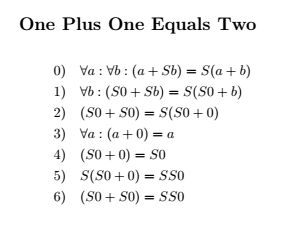## tnt

Simple runtime validated proofs in number theory

### 8 releases(3 stable)

 1.0.2 Nov 18, 2022 Apr 15, 2021 Apr 4, 2021 Mar 30, 2021 Mar 25, 2021

#164 in Math

205KB
1.5K SLoC

# tnt

An implementation of Hofstader's "Typographical Number Theory" from the book Gödel, Escher, and Bach. This crate allows the creation of simple proofs of number theory in propositional logic. It is not a proof assistant, it cannot create a proof or disproof of a given formula, rather the Deduction struct enforces the rules of inference at run time to prevent an invalid proof from being created. Certain nonsensical constructions are also caught at compile time.

A brief explanation is available in the primer.

Once a Deduction is created it can be output in a variety of ways.

Consider the following short proof that 1 + 1 = 2.

use tnt::types::{Term, Variable, Number};
use tnt::deduction::Deduction;
use tnt::axioms::PEANO;

let a = &Variable::new("a");
let b = &Variable::new("b");
let zero = &Number::zero();
let one = &Number::one();

let mut d = Deduction::new("One Plus One Equals Two", PEANO.clone());
d.specification(0, a, one)?;
d.specification(1, b, zero)?;
d.specification(3, a, one)?;
d.successor(4)?;
d.transitivity(2, 5)?;


Using .pretty_print()

0) Aa:Ab:(a+Sb)=S(a+b)
1) Ab:(S0+Sb)=S(S0+b)
2) (S0+S0)=S(S0+0)
3) Aa:(a+0)=a
4) (S0+0)=S0
5) S(S0+0)=SS0
6) (S0+S0)=SS0


\documentclass[fleqn,11pt]{article}
\usepackage{amsmath}
\allowdisplaybreaks
\begin{document}
\section*{One Plus One Equals Two}
\begin{flalign*}
&\hspace{0em}0)\hspace{1em}\forall a:\forall b:(a+Sb)=S(a+b)\\
&\hspace{0em}1)\hspace{1em}\forall b:(S0+Sb)=S(S0+b)\\
&\hspace{0em}2)\hspace{1em}(S0+S0)=S(S0+0)\\
&\hspace{0em}3)\hspace{1em}\forall a:(a+0)=a\\
&\hspace{0em}4)\hspace{1em}(S0+0)=S0\\
&\hspace{0em}5)\hspace{1em}S(S0+0)=SS0\\
&\hspace{0em}6)\hspace{1em}(S0+S0)=SS0\\
\end{flalign*}


Which renders as:The Deduction can also be crudely translated to English with automatic annotations using the .english() method.

0) for all a and b, (a + (b + 1)) = ((a + b) + 1) [axiom]
1) for all b, (1 + (b + 1)) = ((1 + b) + 1) [specification of a to S0 in theorem 0]
2) (1 + 1) = ((1 + 0) + 1) [specification of b to 0 in theorem 1]
3) for all a, (a + 0) = a [axiom]
4) (1 + 0) = 1 [specification of a to S0 in theorem 3]
5) ((1 + 0) + 1) = 2 [successor of theorem 4]
6) (1 + 1) = 2 [transitivity of theorem 2 and theorem 5]


Finally a Deduction may be rendered as an (extremely large) integer by using the .arithmetize() method which reads each theorem as bytes seperated by spaces into a BigUint. This is of no practical use the author is aware of but is relevant to the production of Gödel statements.

The previous theorem corresponds to the number: 1050341303275422378657768361784977847949672579265786753539438511912991722480303393035798790127074435266152493569318923916943104808524883160525578709392832871799037992734723295688338121067685795026664762465244602602804284806503763532291647776

# Future Goals

Currently the .english() method relies a hand-made parser. This should be changed to a crate like pest or nom to avoid bugs.

Better documentation for Deduction.

~5.5–7MB
~133K SLoC